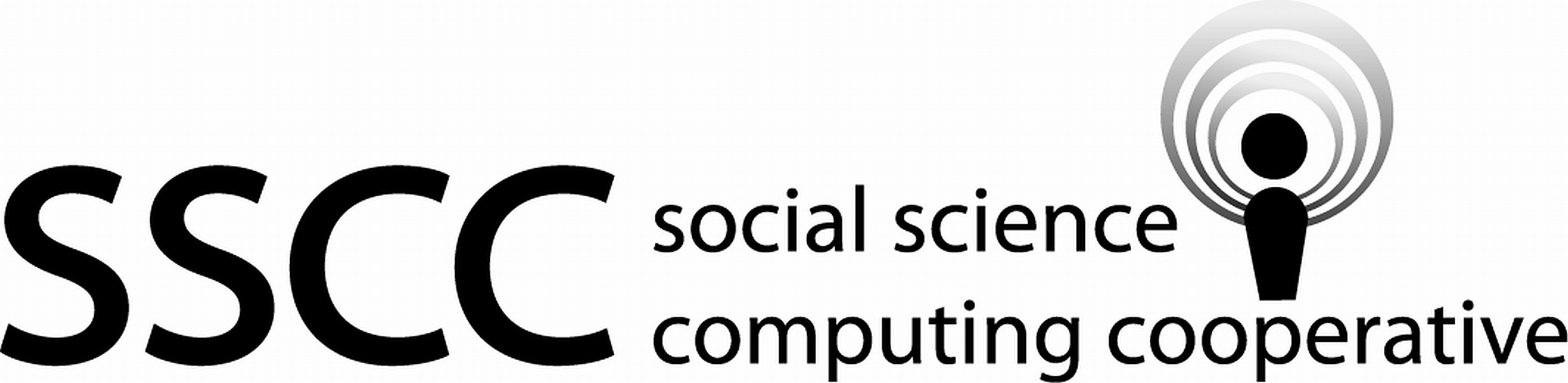Supporting Statistical Analysis for Research

## 4.2 Specifying a model

### 4.2.1 Formula

The response variable and model variables are specified as an R formula. The basic form of a formula is

$response \sim term_1 + \cdots + term_p.$

The tilda, ~, separates the response variable, on the left, from the terms of the model, which are on the right. Terms are are either the main effect of a regressor or the interaction of regressors. Model variables are constructed from terms. For example, a factored regressor, R's implementation of a categorical variable, can be used as term in a model and it results in multiple model variables, one for each non reference level category. There is one coefficient for each model variable and possibly multiple coefficients for a term.

The mathematical symbols, +, -, *, and ^ do not have their normal mathematical meaning in a formula. The + symbol is used to add additional terms to a fromula, The - symbol is used to remove a term from a formula. The decriptions for * and ^ are included with the formula shortcuts below.

Some common terms are

• Numeric regressor: All numeric variable types result in a single continuous model variable.
• Logical regressor: Results in a single indicator, also known as dummy variable, in the model.
• Factored regressor: Results in a set of indicator variables in the model. The first level of the factor is the reference level and is not coded as an indicator variable. All other levels are encoded as indicator variables.
• $$term_1:term_2$$: Creates a term from the interaction between terms $$term_1$$ and $$term_2$$. The : operator does not force the main effect terms, $$term_1$$ and $$term_2$$, into the model.

Some formula short cuts for specifying terms.

• $$term_1*term_2$$: This results in the same interaction term as $$term_1:term_2$$. The * operator forces the main effect terms $$term_1$$ and $$term_2$$ into the model.
• $$(term_1 + \cdots + term_j)$$^$$k$$: Creates a term for each of the $$j$$ terms and all interactions up to order $$k$$ which can be formed from the $$j$$ terms.
• I($$expression$$): The I() function is used when you need to use +, -, *, or ^ as math symbols to construct a term in the formula. This is commonly used to construct a quadratic term.
• poly(x, degree=k): Creates a term which is a jth order polynomial of the regressor x. Poly() constructs k model variables which are orthogonal to each other. A poly term, like a factor, is a single term which translates into multiple model variables. These behaviors, orthogonality of the model variables and grouping the k model variables, are advantages when doing variable selection. These orthogonal model variables are difficult to interpret since they are not on the scale of the original regressors. Therefore it is common practice to use poly for model selection and then rerun the selected model using polynomial terms constructed from the regressor after the variable selection process is complete.
• -1: Removes the intercept from the model. The intercept is included in a model by default in R.

As an example consider the formula, y ~ A + B*C. Where A and B are numeric regressors and C is a categorical regressors with three levels, level1, level2, and level3. This formula results in the following terms and model variables.

• (Intercept): This term is a model constant.
• (Intercept): The constant term.
• A: This term results in a single continuous model variable.
• A: A continuous model variable.
• B: This term results in a single continuous model variable.
• B: A continuous model variable.
• C: This term results in two model variables.
• Clevel2: An indicator variable that represents a change in the intercept.
• Clevel3: An indicator variable that represents a change in the intercept.
• B:C: This term results in two model variables.
• B:Clevel2: An indicator variable that represents a change in the B slope value.
• B:Clevel3: An indicator variable that represents a change in the B slope value.